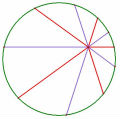# 99華江高中

## 99華江高中

f(n)為n表示成a0．1 + a1．2 + a2．2^2+ a3．2^3+ … 之方法數

4 = 0．1 + 2．2
4 = 2．1 + 1．2

(2)以評量觀點來看，(1)的敘述略有不妥，試說明

ps.第(1)小題15分，第(2)小題6分

[ 本帖最後由 bugmens 於 2010-7-16 05:57 PM 編輯 ]

TOP

 老王 老王發短消息 加為好友 當前離線 2# 大 中 小 發表於 2010-7-16 11:40  只看該作者 提供淺見 (1)如果n是奇數，那麼必定要用一個1， 也就是$$a_0=1$$ $$\displaystyle n=1+a_1\cdot 2+a_2\cdot 2^2+a_3\cdot 2^3+...+a_k\cdot 2^k$$ $$\displaystyle n-1=a_1\cdot 2+a_2\cdot 2^2+a_3\cdot 2^3+...+a_k\cdot 2^k$$ $$\displaystyle \frac{n-1}{2}=a_1\cdot 1+a_2\cdot 2+a_3\cdot 2^2+...+a_k\cdot 2^{k-1}$$ 於是n的一種表示法就對應$$\frac{n-1}{2}$$ 的一種表示法，這是一一對應的，故有 $$\displaystyle f(n)=f(\frac{n-1}{2})$$ (2)如果n是偶數，那麼$$a_0$$可以是0或2 若$$a_0=0$$ $$\displaystyle n=a_1\cdot 2+a_2\cdot 2^2+a_3\cdot 2^3+...+a_k\cdot 2^k$$ $$\displaystyle \frac{n}{2}=a_1\cdot 1+a_2\cdot 2+a_3\cdot 2^2+...+a_k\cdot 2^{k-1}$$ 而若$$a_0=2$$ $$\displaystyle n=2+a_1\cdot 2+a_2\cdot 2^2+a_3\cdot 2^3+...+a_k\cdot 2^k$$ $$\displaystyle \frac{n-2}{2}=a_1\cdot 1+a_2\cdot 2+a_3\cdot 2^2+...+a_k\cdot 2^{k-1}$$ 所以有$$\displaystyle f(n)=f(\frac{n}{2})+f(\frac{n-2}{2})$$ 所以 $$\displaystyle f(401)=f(200)=f(100)+f(99)=f(50)+f(49)+f(49)=f(25)+f(24)+2f(24)$$ $$\displaystyle =f(12)+3f(12)+3f(11)=4f(6)+4f(5)+3f(5)=4f(3)+4f(2)+7f(2)=4f(1)+11f(2)=26$$ 至於有何不妥，我想是 (1)n應該要是大於1的奇數 (2)只給$$\displaystyle f(n)=f(\frac{n-1}{2})$$ ，尚不足以求出$$f(401)$$的值，要多給一點提示較佳吧。 名豈文章著官應老病休飄飄何所似Essential isolated singularity UID261 帖子308 閱讀權限10 在線時間943 小時 註冊時間2009-5-14 最後登錄2014-3-17  查看詳細資料 TOP

#### 附件99華江高中.pdf (173.16 KB)

2010-7-16 17:59, 下載次數: 6244

TOP

 johncai發短消息 加為好友 當前離線 4# 大 中 小 發表於 2010-7-18 19:48  只看該作者 想請教一下1,6,7題 謝謝! 順便問一下第7題Z的機率是甚麼意思呢? UID535 帖子79 閱讀權限10 在線時間299 小時 註冊時間2010-5-30 最後登錄2021-4-20  查看詳細資料 TOP
 weiye 瑋岳發短消息 加為好友 當前離線 5# 大 中 小 發表於 2010-7-18 20:39  只看該作者 第 1 題 令 $$A(2,-2), B(12,1), C(x,\log x)$$ $$\left|\overline{AC}-\overline{AB}\right|\leq \overline{BC}=\sqrt{109}.$$ 第 6 題 目前只有想到很醜陋的硬算， 令 $$f(x)=ax^3+bx^2+cx+d$$， 由 $$f(1)=2,\,f(2)=5,\,f(3)=10$$， 可得 $$\displaystyle a=\frac{1-d}{6},\,b=d,\,c=\frac{11-11d}{6}$$， 再帶入 $$b^2-3ac=0$$，可得 $$d$$ 之值。 故，可得 $$f(x)$$，亦可得 $$f(4).$$ 第 7 題 $$Z$$ 分配：平均數為 $$0$$ 且標準差為 $$1$$ 的常態分配。 $$75$$ 分以上所佔比例為 $$\displaystyle\frac{12}{300}=0.04=0.5-0.46$$ 因為測驗分數成常態分配， 所以 $$75$$ 分＝平均分數＋$$1.75$$個標準差 。 故，此次測驗平均分數為 $$75-8\times1.75=61$$。 UID1 帖子2286 閱讀權限200 在線時間7812 小時 註冊時間2006-3-5 最後登錄2021-11-26  查看詳細資料 TOP
 liengpi發短消息 加為好友 當前離線 6# 大 中 小 發表於 2010-7-20 00:19  只看該作者 補一下初試通過最低錄取分數  64分   雖然我進了複試 可是我面試時被電的非常的慘烈 UID291 帖子22 閱讀權限10 在線時間77 小時 註冊時間2009-6-17 最後登錄2011-7-13  查看詳細資料 TOP
 arend發短消息 加為好友 當前離線 7# 大 中 小 發表於 2010-7-20 01:37  只看該作者 請問第8題的答案為何? 謝謝 UID227 帖子374 閱讀權限10 在線時間729 小時 註冊時間2009-2-22 最後登錄2021-10-2  查看詳細資料 TOP
 arend發短消息 加為好友 當前離線 8# 大 中 小 發表於 2010-7-20 01:45  只看該作者 請問瑋岳老師 您第三題的解中寫到 b^2-3ac=0 跟原題中恰有一水平切線 怎麼個解釋 謝謝 UID227 帖子374 閱讀權限10 在線時間729 小時 註冊時間2009-2-22 最後登錄2021-10-2  查看詳細資料 TOP
 weiye 瑋岳發短消息 加為好友 當前離線 9# 大 中 小 發表於 2010-7-20 08:12  只看該作者 $$f(x)=ax^3+bx^2+cx+d\Rightarrow f\,'(x)=3ax^2+2bx+c$$ $$\Rightarrow f\,'(x)=0$$ 的判別式為 $$\left(2b\right)^2-4\cdot\left(3a\right)\cdot c=4\left(b^2-3ac\right)$$ 所以 case 1: $$y=f(x)$$ 有兩條水平切線$$\Leftrightarrow f\,'(x)=0$$ 有兩相異實根$$\Leftrightarrow b^2-3ac>0.$$ case 2: $$y=f(x)$$ 恰有一條水平切線$$\Leftrightarrow f\,'(x)=0$$ 有兩相等實根$$\Leftrightarrow b^2-3ac=0.$$ case 3: $$y=f(x)$$ 無水平切線$$\Leftrightarrow f\,'(x)=0$$ 無實根$$\Leftrightarrow b^2-3ac<0.$$ UID1 帖子2286 閱讀權限200 在線時間7812 小時 註冊時間2006-3-5 最後登錄2021-11-26  查看詳細資料 TOP
 arend發短消息 加為好友 當前離線 10# 大 中 小 發表於 2010-7-20 15:12  只看該作者 謝謝瑋岳老師 把他想成有反曲點 所以用二次微分等於0,好複雜 UID227 帖子374 閱讀權限10 在線時間729 小時 註冊時間2009-2-22 最後登錄2021-10-2  查看詳細資料 TOP
﻿